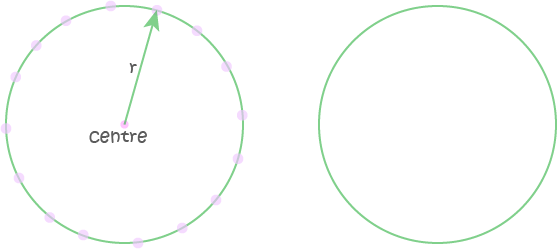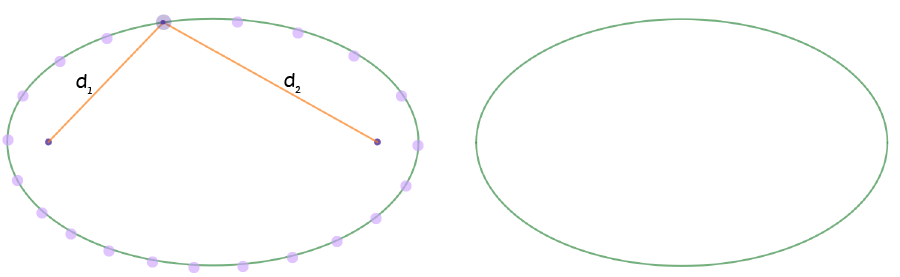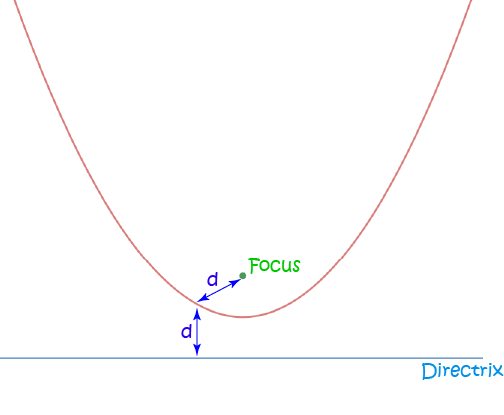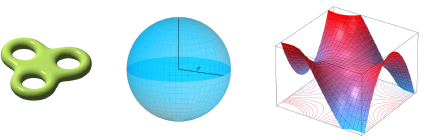Pull Down & Release to Refresh

# The Set of All Points That ... (Locus)

A set is a collection of objects that have some property in common.

Mathematicians often talk about the set of all points that have a certain property. The technical term for this is a Locus. The set of points satisfying a property often forms a line, a surface or a curve. We can then say that the locus is the path taken by a point that moves so that it satisfies the property.

Let's have a look at some examples.

## A Straight Line

A straight line can be thought of as the set of all points that satisfy a certain equation, or the path taken by a point that moves so that it is the same distance from two other points. If we fill in a few of these points, then a few more, and then a few more, we start to see that the locus of these points is a straight line, as shown in the picture below:## A Circle

When you draw a circle with a pair of compasses, you set a radius, stick the end of your compasses into the paper, and swing your pencil around so that it traces out the set of all points that are a fixed distance (the radius) away from a fixed point (the centre) of the circle. So, the circle is an example of a locus as well. To confirm this, you could plot a number of points, and join them up to form the circle, as shown in the picture below:## An Ellipse

An ellipse is a bit like a squashed circle. Instead of a fixed central point, we have two central points called foci. The ellipse is the locus of a point that moves so that its distances from two fixed points, called the foci, add up to some fixed constant. No matter where a point is on the ellipse, the distances $d_1$ of the point from the first focus and $d_2$ from the second focus add up to give a constant:

$d_1 + d_2 = \text{constant}$.
To confirm this, you could plot a number of points, and join them up to form the ellipse, as shown in the picture below:## A Parabola

A parabola, e.g. the graph of $y = x^2$ is an example of a locus too. It is the locus of a point that moves so that its distance from a fixed point, called the focus, is equal to its distance from a fixed line, called the directrix, as shown in the picture below:## In 3-Dimensional Space

Can we talk about a locus in 3-D space as well? Of course we can. We can come up with all sorts of weird and wonderful surfaces. Here are a few examples:The middle one is a sphere, of course. Its description as a locus is easy to understand: like the circle, it is the locus of a point that moves so that its distance from a fixed point, called the centre is equal to a fixed constant, called the radius. The others can be described as sets of points as well, but their descriptions are a bit too complicated to give in this article.

### Description

coordinate geometry is a branch of geometry where the position of the points on the plane is defined with the help of an ordered pair of numbers also known as coordinates. In this tutorial series, you will learn about vast range of topics such as Cartesian Coordinates, Midpoint of a Line Segment etc

### Audience

year 10 or higher, several chapters suitable for Year 8+ students.

### Learning Objectives

Explore topics related to Coordinates Geometry

Author: Subject Coach
You must be logged in as Student to ask a Question.